CATEGORIES:

# Vector Product and Its Properties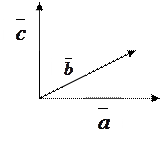Consider two vectors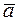and:

Definition. The vector product of two vectorsandis a vector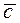, satisfying the following conditions:

(1) the absolute value of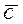equals the product of the absolute values of the two given vectors and the sine of the angle between them: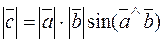; (*)

(2) the vector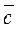is perpendicular to both vectorsand:;

(3) the three vectors,andconstitute a right triple of vectors (that is, looking from the tail of, we see that the shorter rotation fromtois carried out anticlockwise). The vector product ofandis denoted by.

1.The absolute value of the vector product of two vectors is equal to the area of the parallelogram spanned by these vectors: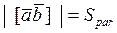.

2. The vector product is anticommutative, i.e.,.

3. To multiply a vector product by a number l, it is suffices to multiply one of the vectors by this number (without proof):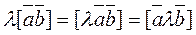.

4. Vector product is associative: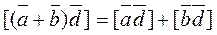.

5. The vector product of collinear vectors is equal to zero, and vice versa, if the vector product of two vectors is zero, then these vectors are collinear.

Date: 2015-01-02; view: 1536

 <== previous page | next page ==> The direction of a vector. Let us find the angle between two vectors and . | Consider vectors
doclecture.net - lectures - 2014-2023 year. Copyright infringement or personal data (0.006 sec.)